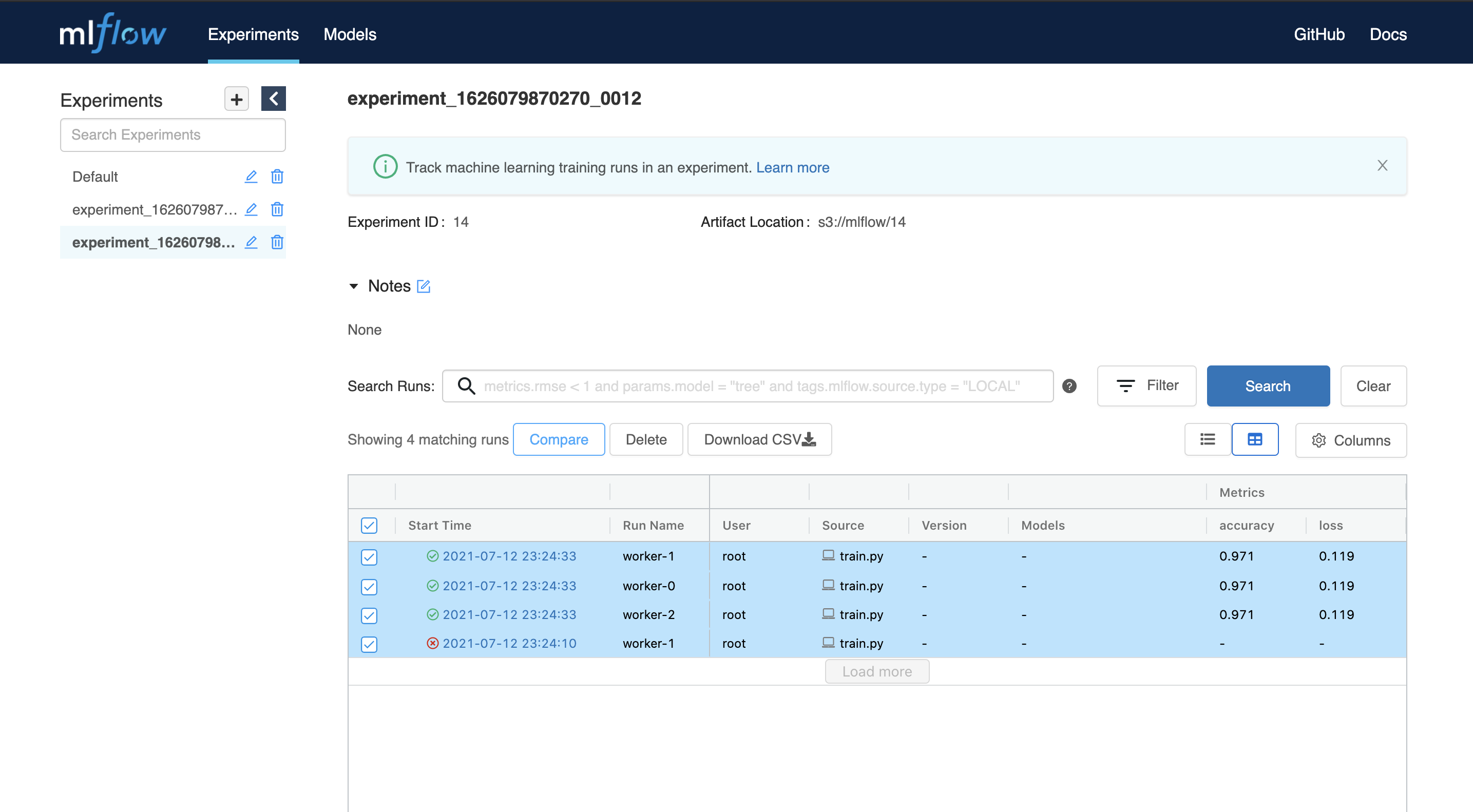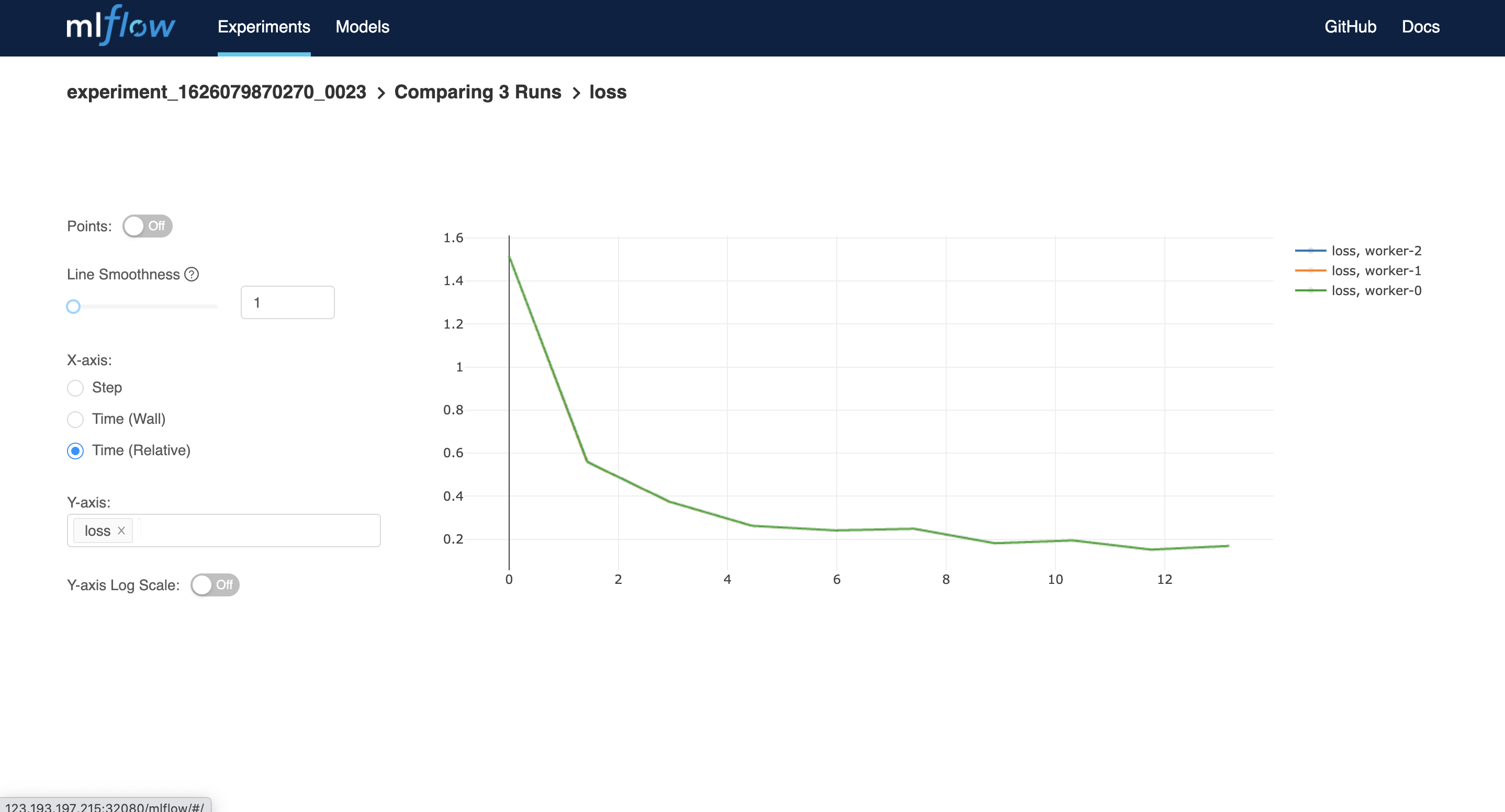Version: 0.6.0

# Quickstart

This document gives you a quick view on the basic usage of Submarine platform. You can finish each step of ML model lifecycle on the platform without messing up with the troublesome environment problems.

## Installation​

### Prepare a Kubernetes cluster​

1. Prerequisite
1. Start minikube cluster
``\$ minikube start --vm-driver=docker --cpus 8 --memory 4096 --kubernetes-version v1.15.11``

### Launch submarine in the cluster​

1. Clone the project
``\$ git clone https://github.com/apache/submarine.git``
1. Install the resources by helm chart
``\$ cd submarine\$ helm install submarine ./helm-charts/submarine``

1. Use kubectl to query the status of pods
``\$ kubectl get pods``
1. Make sure each pod is `Running`
``NAME                                              READY   STATUS    RESTARTS   AGEnotebook-controller-deployment-5d4f5f874c-vwds8   1/1     Running   0          3h33mpytorch-operator-844c866d54-q5ztd                 1/1     Running   0          3h33msubmarine-database-674987ff7d-r8zqs               1/1     Running   0          3h33msubmarine-minio-5fdd957785-xd987                  1/1     Running   0          3h33msubmarine-mlflow-76bbf5c7b-g2ntd                  1/1     Running   0          3h33msubmarine-server-66f7b8658b-sfmv8                 1/1     Running   0          3h33msubmarine-tensorboard-6c44944dfb-tvbr9            1/1     Running   0          3h33msubmarine-traefik-7cbcfd4bd9-4bczn                1/1     Running   0          3h33mtf-job-operator-6bb69fd44-mc8ww                   1/1     Running   0          3h33m``

### Connect to workbench​

1. Port-forwarding
``# using port-forwarding\$ kubectl port-forward --address 0.0.0.0 service/submarine-traefik 32080:80``
1. Open `http://0.0.0.0:32080`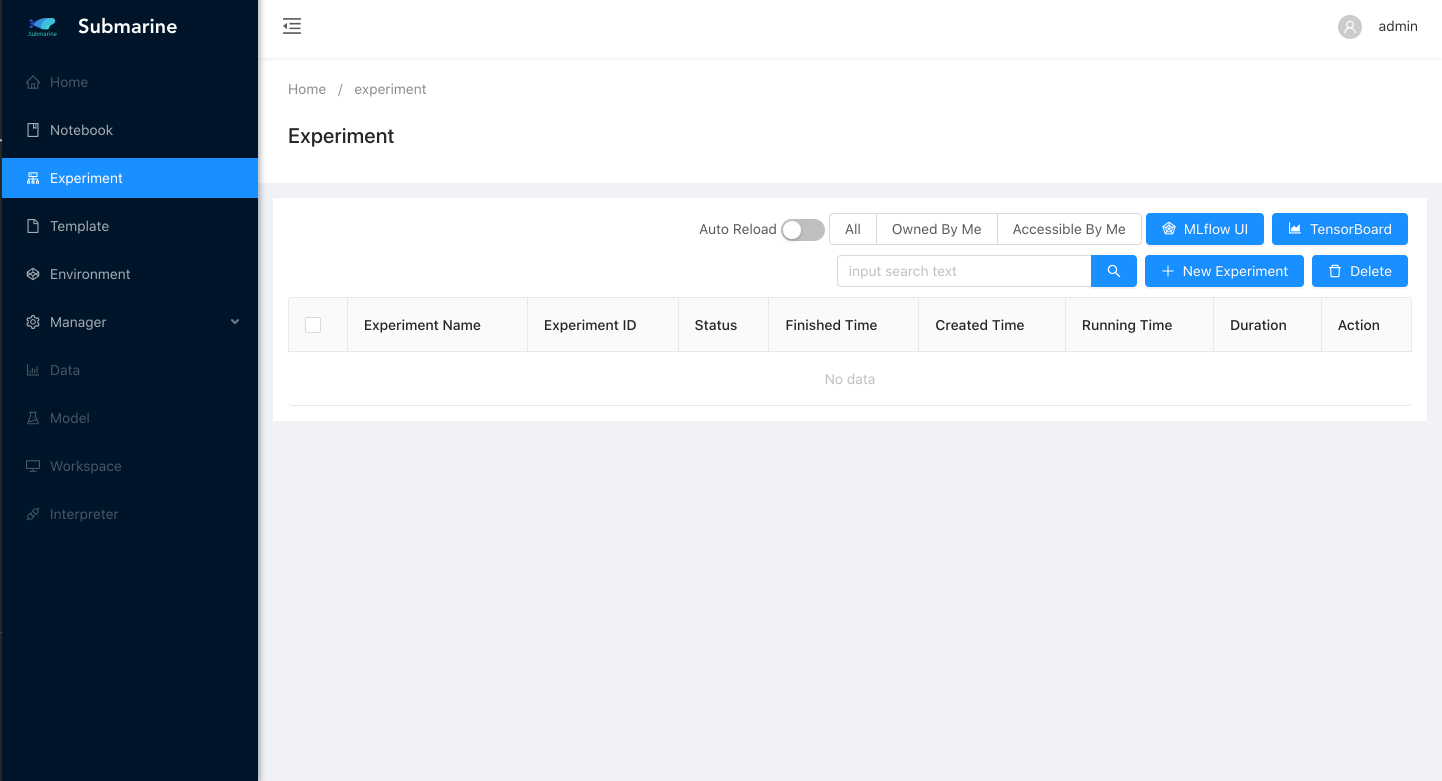## Example: Submit a mnist distributed example​

We put the code of this example here. `train.py` is our training script, and `build.sh` is the script to build a docker image.

### 1. Write a python script for distributed training​

Take a simple mnist tensorflow script as an example. We choose `MultiWorkerMirroredStrategy` as our distributed strategy.

``"""./dev-support/examples/quickstart/train.pyReference: https://github.com/kubeflow/tf-operator/blob/master/examples/v1/distribution_strategy/keras-API/multi_worker_strategy-with-keras.py"""import tensorflow_datasets as tfdsimport tensorflow as tffrom tensorflow.keras import layers, modelsfrom submarine import ModelsClientdef make_datasets_unbatched():  BUFFER_SIZE = 10000  # Scaling MNIST data from (0, 255] to (0., 1.]  def scale(image, label):    image = tf.cast(image, tf.float32)    image /= 255    return image, label  datasets, _ = tfds.load(name='mnist', with_info=True, as_supervised=True)  return datasets['train'].map(scale).cache().shuffle(BUFFER_SIZE)def build_and_compile_cnn_model():  model = models.Sequential()  model.add(      layers.Conv2D(32, (3, 3), activation='relu', input_shape=(28, 28, 1)))  model.add(layers.MaxPooling2D((2, 2)))  model.add(layers.Conv2D(64, (3, 3), activation='relu'))  model.add(layers.MaxPooling2D((2, 2)))  model.add(layers.Conv2D(64, (3, 3), activation='relu'))  model.add(layers.Flatten())  model.add(layers.Dense(64, activation='relu'))  model.add(layers.Dense(10, activation='softmax'))  model.summary()  model.compile(optimizer='adam',                loss='sparse_categorical_crossentropy',                metrics=['accuracy'])  return modeldef main():  strategy = tf.distribute.experimental.MultiWorkerMirroredStrategy(      communication=tf.distribute.experimental.CollectiveCommunication.AUTO)  BATCH_SIZE_PER_REPLICA = 4  BATCH_SIZE = BATCH_SIZE_PER_REPLICA * strategy.num_replicas_in_sync  with strategy.scope():    ds_train = make_datasets_unbatched().batch(BATCH_SIZE).repeat()    options = tf.data.Options()    options.experimental_distribute.auto_shard_policy = \        tf.data.experimental.AutoShardPolicy.DATA    ds_train = ds_train.with_options(options)    # Model building/compiling need to be within `strategy.scope()`.    multi_worker_model = build_and_compile_cnn_model()  class MyCallback(tf.keras.callbacks.Callback):    def on_epoch_end(self, epoch, logs=None):      # monitor the loss and accuracy      print(logs)      modelClient.log_metrics({"loss": logs["loss"], "accuracy": logs["accuracy"]}, epoch)  with modelClient.start() as run:    multi_worker_model.fit(ds_train, epochs=10, steps_per_epoch=70, callbacks=[MyCallback()])if __name__ == '__main__':  modelClient = ModelsClient()  main()``

### 2. Prepare an environment compatible with the training​

Build a docker image equipped with the requirement of the environment.

``\$ ./dev-support/examples/quickstart/build.sh ``

### 3. Submit the experiment​

1. Open submarine workbench and click `+ New Experiment`

2. Fill the form accordingly. Here we set 3 workers.

1. Step 1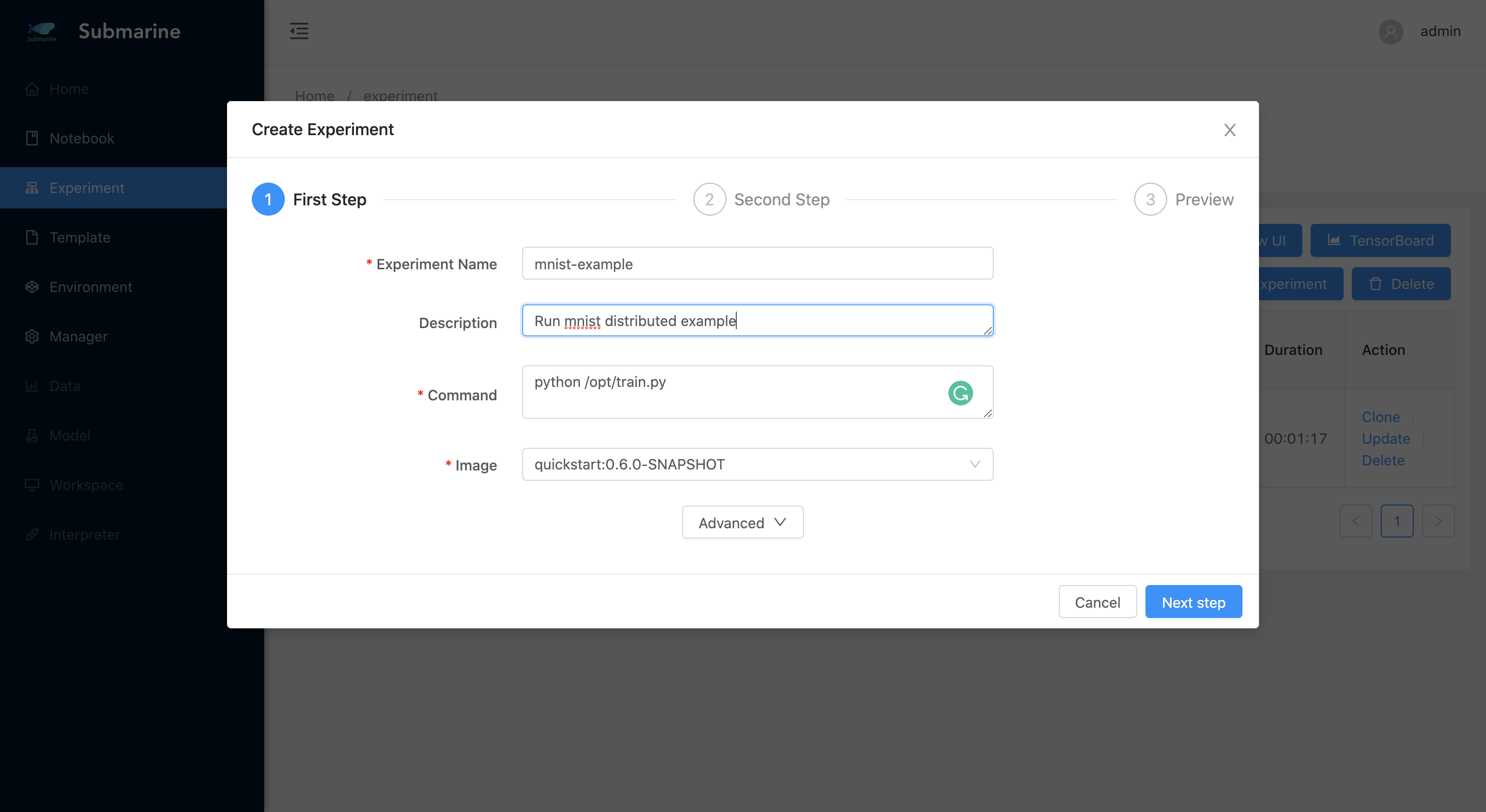2. Step 2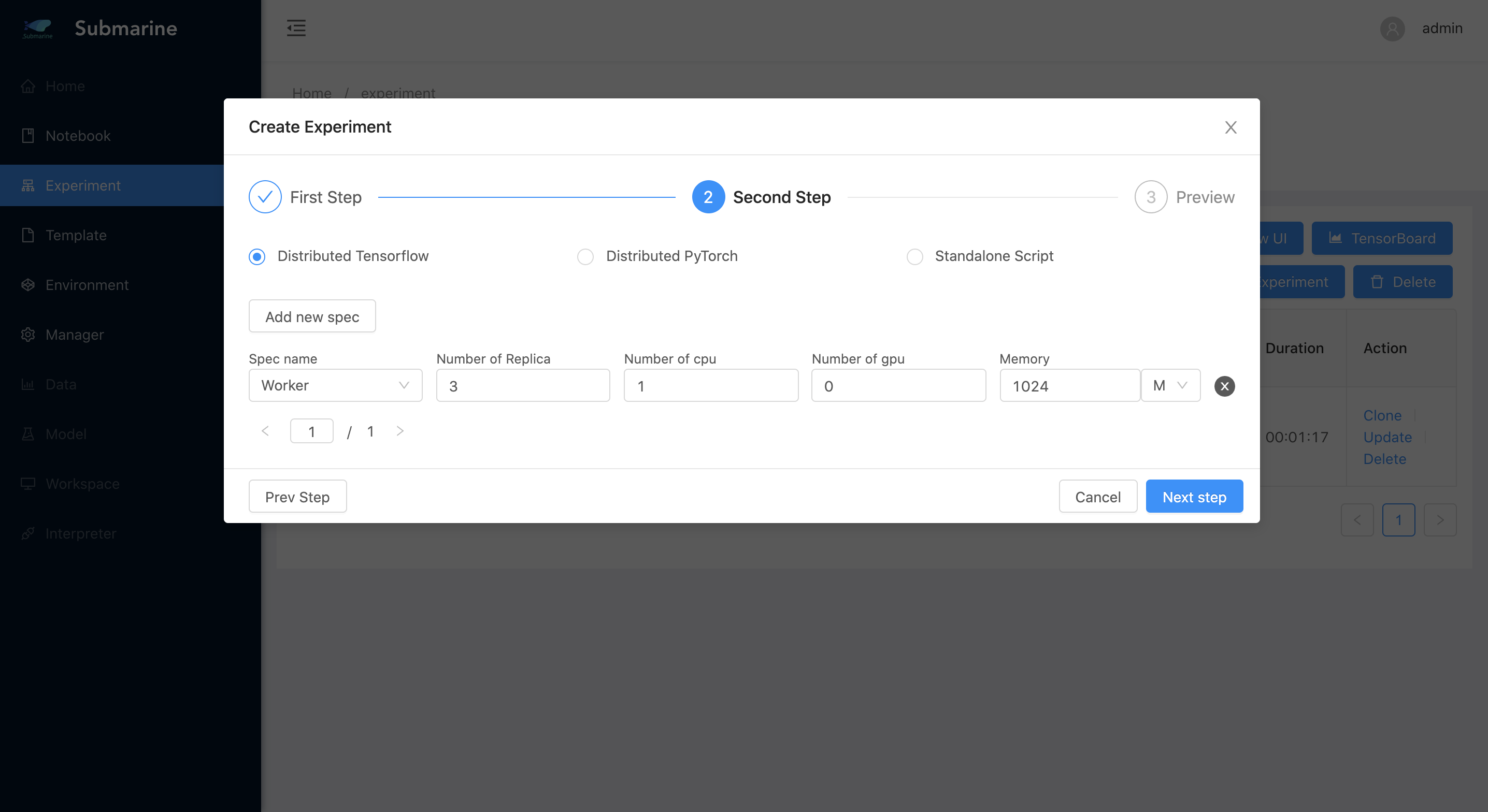3. Step 3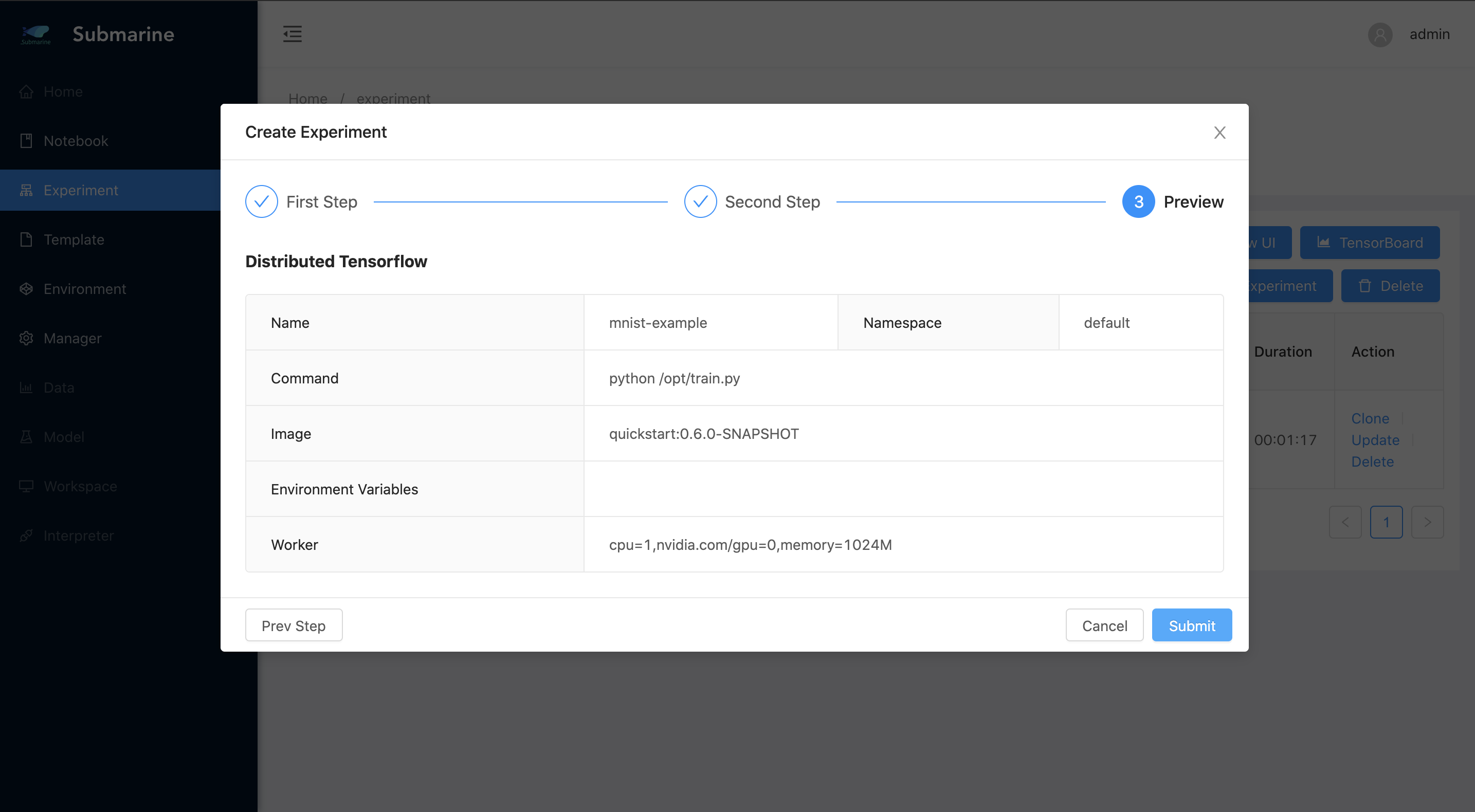4. The experiment is successfully submitted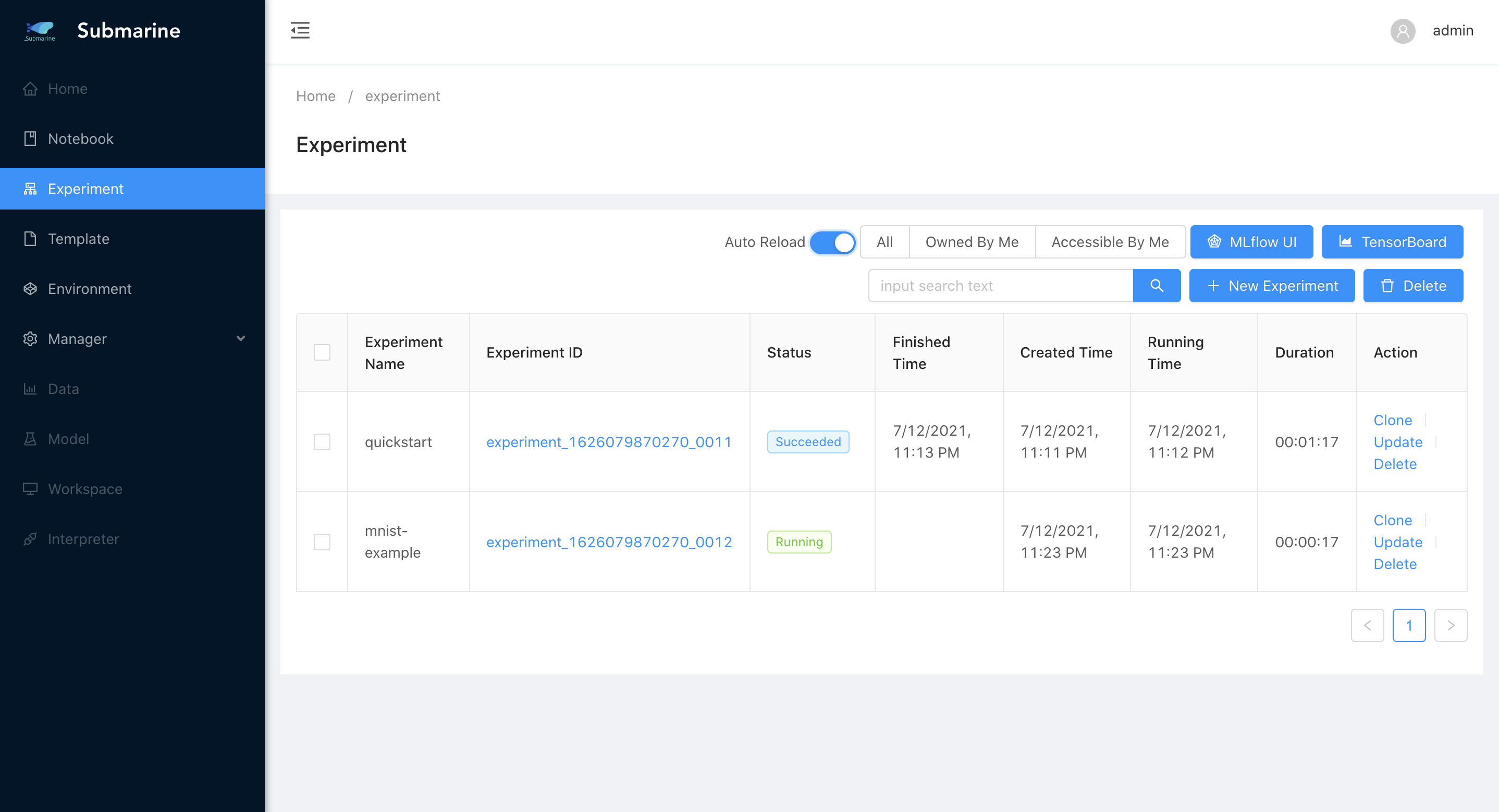### 4. Monitor the process (modelClient)​

1. In our code, we use `modelClient` from `submarine-sdk` to record the metrics. To see the result, click `MLflow UI` in the workbench.

2. To compare the metrics of each worker, you can select all workers and then click `compare`